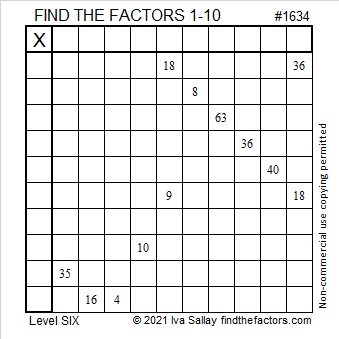# 1634 Be Prepared for April Showers

Contents

### Today’s Puzzle:

If you learn the multiplication and division facts in a standard multiplication table, you will be prepared to solve this somewhat tricky April Shower puzzle. You will also be able to solve MANY other mathematical challenges. Use logic to solve it, not guess and check, and it will be much less challenging to find the missing factors.### Factors of 1634:

• 1634 is a composite number.
• Prime factorization: 1634 = 2 × 19 × 43.
• 1634 has no exponents greater than 1 in its prime factorization, so √1634 cannot be simplified.
• The exponents in the prime factorization are 1, 1, and 1. Adding one to each exponent and multiplying we get (1 + 1)(1 + 1)(1 + 1) = 2 × 2 × 2 = 8. Therefore 1634 has exactly 8 factors.
• The factors of 1634 are outlined with their factor pair partners in the graphic below.### More about the Number 1634:

1634 is part of exactly two Pythagorean triples. Here are the formulas you can use to calculate those two triples:
2(817)(1), 817² – 1², 817² + 1, and
2(43)(19), 43² – 19², 43² + 19².

Do you see the factors of 1634 prominently displayed in those formulas?

This site uses Akismet to reduce spam. Learn how your comment data is processed.